# Algebra 1 : How to factor a trinomial

## Example Questions

1 3 Next →

### Example Question #21 : Trinomials

Factor the following trinomial: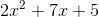Possible Answers: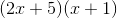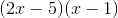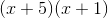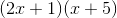Correct answer:Explanation:

To factor the trinomial, its general form given by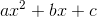, we must find factors of the product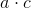that when added together give usFor our trinomial,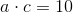and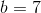. The two factors that fit the above rule areand, because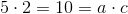and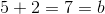.

Using the two factors, we can rewrite the term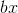as a sum of the two factors added together and multiplied by x: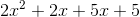Now, we must factor by grouping, which means we group the first two terms, and the last two terms, and factor them: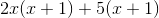Note that after we factored the two groups of terms, what remained inside the parentheses is identical for the two groups.

Simplifying further, we get, which is our final answer.

### Example Question #22 : How To Factor A Trinomial

Which of the following is a perfect square trinomial?

Possible Answers:Correct answer:Explanation:

A perfect square trinomial takes the form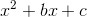,

where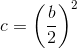Since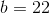, forto be a perfect square,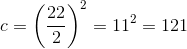.

This makes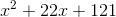the correct choice.

1 3 Next →

### All Algebra 1 Resources